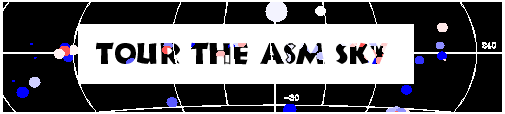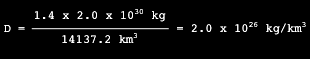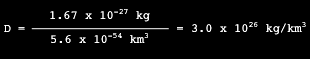# National Aeronautics and Space Administration

## For Educators### Calculating a Neutron Star's Density

A typical neutron star has a mass between 1.4 and 5 times that of the Sun. It cannot be more massive than this or gravity will overwhelm it and it will become a black hole! The radius of a neutron star may be between 10 and 20 kilometers.

Suppose we have a neutron star that has a radius of 15 kilometers and a mass of 1.4 times that of the Sun. (The Sun's mass is 2.0 x 1030 kilograms.) What is the neutron star's density?

Remember, density (D) = mass ÷ volume and the volume (V) of a sphere is (4/3)r3.

V = (4/3)(15.0 km)3
=14137.2 km3Can we compare the density of a neutron star, which is made up of neutrons, to the density of a single neutron?

By shooting particles at a neutron, we can find out its cross-sectional area, and thus its radius and volume. From this we find that the volume of a neutron is approximately 5.6 x 10-54 km3 and the mass of a neutron is 1.67 x 10-27 kg, calculate the density.